Mathematics
Easy

Question

# If the sum is 10 when two fair dice are tossed then the probability is__________.

##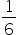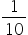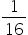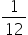Hint:

## The correct answer is:### Total number outcomes = 36[ (1,1), (1,2), (1,3), (1,4), (1,5), (1,6)(2,1), (2,2), (2,3), (2,4), (2,5), (2,6)(3,1), (3,2), (3,3), (3,4), (3,5), (3,6)(4,1), (4,2), (4,3), (4,4), (4,5), (4,6)(5,1), (5,2), (5,3), (5,4), (5,5), (5,6)(6,1), (6,2), (6,3), (6,4), (6,5), (6,6)]Possible outcomes = 3Possibility =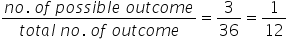So, if the sum is 10 when two fair dice are tossed then the probability is#### With Turito Foundation.#### Get an Expert Advice From Turito.